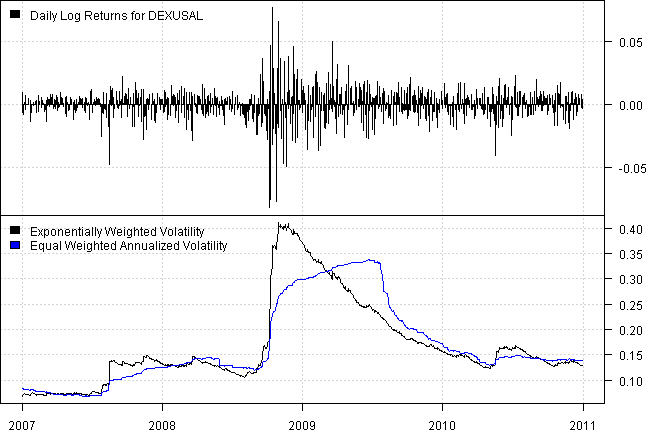# Exponentially Weighted Volatility using RCPP

The Exponentially Weighted Volatility is a measure of volatility that put more weight on the recent observations. We will use following formula to compute the Exponentially Weighted Volatility:

S[t]^2 = SUM (1-a) * a^i * (r[t-1-i] - rhat[t])^2, i=0 … inf

where rhat[t] is the corresponding the Exponentially Weighted mean

rhat[t] = SUM (1-a) * a^i * r[t-1-i], i=0 … inf

## For reference on the computations of Exponentially Weighted Volatility please check:

The above formula depends on the full price history at each point in time and took a while to compute. Hence, I want to share how Rcpp and RcppParallel helped to reduce computation time.

I will use a historic dataset of the Foreign Exchange Rates as my testing data.

First we compute average rolling volatility

 “Elapsed time is 0.17 seconds\n”

Next, let’s code the Exponentially Weighted logic

 “Elapsed time is 106.16 seconds\n”

It took a while to execute this code. Fortunately, the code can easily run in parallel. Following is the RcppParallel version.

 “Elapsed time is 14.65 seconds\n”

Great, the running time is more reasonable. Next let’s visualize the impact of using the Exponentially Weighted VolatilityAs expected, the Exponentially Weighted Volatility puts more weight on most recent observations and is a more reactive risk measure.

For your convenience, the 2016-04-10-Exponentially-Weighted-Volatility-RCPP post source code.